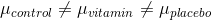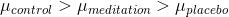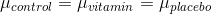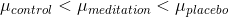AP Statistics : Significance Logic and Establishing Hypotheses

Example Questions

Example Question #1 : How To Establish An Alternate Hypothesis

A researcher wants to investigate the claim that taking vitamins will help a student study longer. First, the researcher collects 32 students who do not take vitamins and determines their time spent studying. Then, the 32 students are given a vitamin for 1 week. After 1 week of taking vitamins, students are again tested to determine their time spent studying. Which of the following is the correct alternative hypothesis?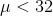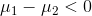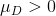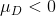Explanation:

Because the same students are tested twice, this is a paired study, therefore we must use a hypothesis appropriate for a paired t-test.  The hypothesis for a paired t-test regards the average of the differences between before and after treatment, called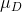. We are testing the claim that vitamins increase study time, therefore this will be the alternative hypothesis. This means that the difference between control – treatment is greater than zero, since the average study time for vitamin users would be greater than that of the control.

Example Question #2 : How To Establish An Alternate Hypothesis

A study would like to determine whether meditation helps students improve focus time. They know that the average focus time of an American 4th grader is 23 minutes. They then recruit 50 meditators and calculate their average focus time. What is the appropriate alternative hypothesis for this study?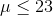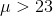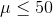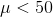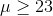Explanation:

Because we are comparing one sample to a known mean, the hypothesis takes the form of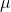compared to the known value.  We would like to test the claim that meditating will increase focus time, so this will be the alternative hypothesis. Therefore, the alternative thatis greater than the average of 23 minutes.

Example Question #31 : Inference

A study would like to determine whether meditation helps students increase focus time. They used a control group of 30 students and compared their focus time to a group of 30 meditating students and compared their average time spent meditating.  What is the appropriate alternative hypothesis for this study?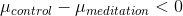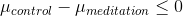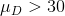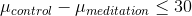Explanation:

Because we are comparing two samples, the hypothesis takes the form of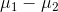. The study is testing the claim that meditation helps increase focus time, so this will be our alternative hypothesis. Our alternative hypothesis in words is that the difference between control and meditation groups is less than zero. That is because we think average time for meditators will be greater than the control group’s average.

Example Question #1 : How To Establish A Null Hypothesis

Jimmy thinks that Josh cannot shoot more than 50 points on average in a game. Josh disputes this claim and tells Jimmy that he is going to play 10 games and prove him wrong. What is the null hypothesis?

Josh cannot shoot exactly 50 points.

Josh cannot shoot less than 50 points.

Josh cannot shoot more than 50 points.

Josh cannot play 10 games.

Josh cannot shoot more than 50 points.

Explanation:

The null hypothesis is what we intend to either reject or fail to reject using our sample data. In this case, the null hypothesis is that Josh cannot shoot more than 50 points on average, and Josh's performance in 10 games are the sample data we use to assess this hypothesis.

Example Question #2 : How To Establish A Null Hypothesis

A student is beginning an analysis to determine whether there is a relationship between temperatures and traffic accidents.  The student is trying to articulate a null hypothesis for the study.  Which of the following is an acceptable null hypothesis?

There is a negative relationship between temperatures and traffic accidents

No variable can accurately predict whether traffic accidents will increase

Traffic accidents increase as temperatures decrease

There is no relationship between temperatures and frequency of traffic accidents

There is a positive relationship between temperatures and traffic accidents

There is no relationship between temperatures and frequency of traffic accidents

Explanation:

The null hypothesis is the default hypothesis and predicts that there is no relationship between the variables in question.  Each of the incorrect answer choices here either predicts a relationship between variables or makes a broad assertion that includes much more than the variables in question.

Example Question #3 : How To Establish A Null Hypothesis

A statistician has determined that she will reject the null hypothesis if she can have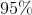confidence that there is a statistically significant relationship between the variables in question. She conducts a statistical analysis and obtains a p value of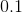.  Should the statistician reject the null hypothesis?

Maybe

Yes

Not enough information to make a decision.

No

Conditionally

No

Explanation:

The statistician has determined that she will only reject the null hypothesis if she has 95% confidence that there is a relationship between variables.

To have this level of confidence, the statistician must obtain a p value of 0.05 or lower.

Therefore, she should not reject the null hypothesis since 0.1 is greater that 0.05.

Example Question #4 : How To Establish A Null Hypothesis

The Environmental Protection Agency (EPA) wants to test the pollution level of the Colorado River. If the pollution level is too high, the water will be stopped from going into drinking water pipelines. The EPA randomly chooses different spots along the river to collect water samples from, and then tests the samples for their pollution levels. Which of the following decisions would result from the type I error?

Keeping the drinking water pipelines open when the pollution levels are higher than the allowed limit.

Closing the drinking water pipelines when the pollution levels are higher than the allowed limit.

Closing the drinking water pipelines because of the endangered frog population.

Closing the drinking water pipelines for the river when the pollution levels are within the allowed limit.

Keeping the drinking water pipelines open when the pollution levels are within the allowed limit.

Closing the drinking water pipelines for the river when the pollution levels are within the allowed limit.

Explanation:

The hypotheses tested here are: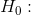The true mean pollution level is within the allowed limit.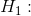The true mean pollution level is higher than the allowed limit.

The type I error occurs when the null hypothesis is rejected even though it is actually true. In this case, the type I error would be deciding that the mean pollution levels are higher than the allowed limit and closing the drinking water pipelines.

Example Question #5 : How To Establish A Null Hypothesis

A study would like to determine whether meditation helps students improve focus time. They know that the average focus time of an American 4th grader is 23 minutes. They then recruit 50 meditators and calculate their average focus time. What is the appropriate null hypothesis for this study?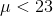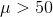Explanation:

Because we are comparing one sample to a known mean, the hypothesis iscompared to the known average, 23.  We would like to test the claim that meditating will increase focus time, therefore our null hypothesis must include all other outcomes. Therefore, the null is that Mu is less than or equal to 23.

Example Question #6 : How To Establish A Null Hypothesis

A researcher wants to determine whether there is a significant linear relationship between time spent meditating and time spent studying. What is the appropriate null hypothesis for this study?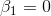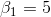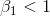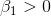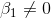Explanation:

This question is about a linear regression between time spent meditating and time spent studying. Therefore, the hypothesis is regarding Beta1, the slope of the line. We are testing a non-directional or bi-directional claim that the relationship is significant. Therefore, the null hypothesis is that the relationship is not significant, meaning the slope of the line is equal to zero.

Example Question #7 : How To Establish A Null Hypothesis

A researcher wants to compare 3 different treatments to see if any of the treatments affects study time. The three treatments studied are control group, a group given vitamins, and a group given a placebo.  They found that the average time spent studying with control students was 2 hours, with students given vitamins it was 3 hours, and with placebos students studied 5 hours. Which of the following is the correct null hypothesis?